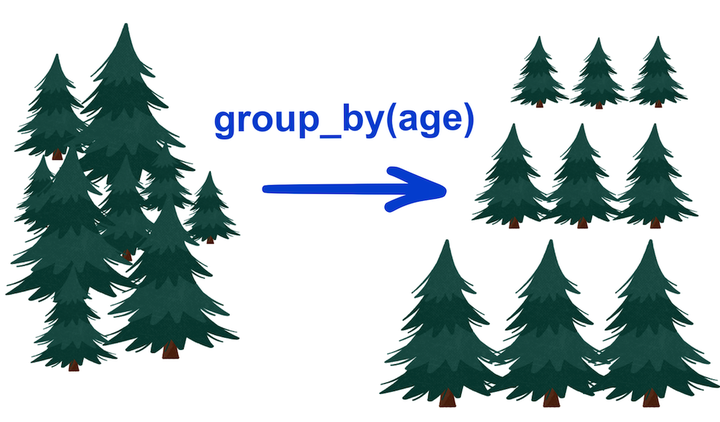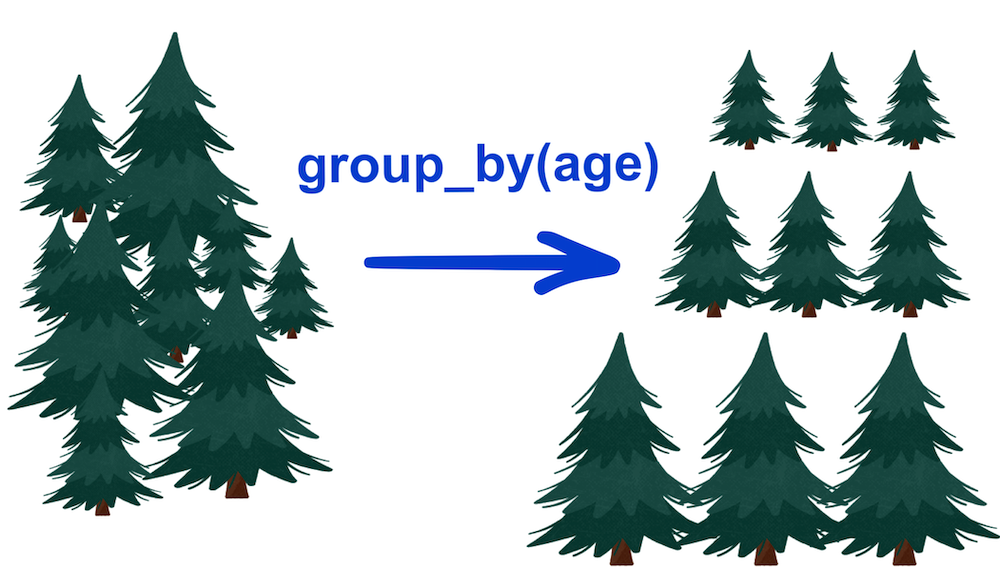# How to use the group_by function with your ecological data

How to use group_by() with other dplyr functions for ecological data wrangling like a proIn scientific data and experiments, we often have groups of subjects between which we want to compare an observed response. For example, we might want to compare the growth rates of plants under different light treatments. Or maybe we want to compare CO² emissions of different countries over time. Each of these scenarios requires you to group your data based on a certain variable before you can compare any kind of statistic such as mean, minimum, or maximum.

In this tutorial, I’m going to discuss how to use a handy function called `group_by()`, which allows you to do what I just described.`group_by()` is part of the `dplyr` package, so we’ll load that up first. Remember that if you haven’t used or installed the package before, you need to run `install.packages("dplyr")` before loading it in your script. Let’s also load up a data set that comes with R, called `Loblolly`.

``````# Load package
library(dplyr)
``````
``````# Load data
data(Loblolly)

# View data
``````
``````##    height age Seed
## 1    4.51   3  301
## 15  10.89   5  301
## 29  28.72  10  301
## 43  41.74  15  301
## 57  52.70  20  301
## 71  60.92  25  301
``````

`Loblolly` describes the height of Loblolly pine trees at different ages. “Height” is given in feet, “age” is given in years, and “seed” is a unique identifier for each tree.

### How to use group_by() and summarise()

Let’s say we want to see the average height of loblolly pine trees within each of the age groups. To do that, we need to group our data by the variable “age”. We use the `group_by()` function like this: `group_by(data, column)`.

``````# Group the Loblolly data by tree age
group_by(Loblolly, age)
``````
``````## # A tibble: 84 × 3
## # Groups:   age 
##    height   age Seed
##     <dbl> <dbl> <ord>
##  1   4.51     3 301
##  2  10.9      5 301
##  3  28.7     10 301
##  4  41.7     15 301
##  5  52.7     20 301
##  6  60.9     25 301
##  7   4.55     3 303
##  8  10.9      5 303
##  9  29.1     10 303
## 10  42.8     15 303
## # … with 74 more rows
``````

When we do this, our data look the same. But behind the scenes, R makes note of how we want to group our data and returns a table that is grouped accordingly. In fact, our data look the same aside from the `Groups: age ` labeled at the top of the table. However, after grouping the data, we can now apply functions that calculate summary statistics within each group using the function `summarize()`, or `summarise()` (the spelling depends on if you use British or American English).

`summarise()` can be used like so: `summarise(data, new_column_name = function(column_to_evaluate))`.

So if we wanted to summarize mean heights of trees, it would look like `summarise(Loblolly, avgheight = mean(height))`.

``````# Group the Loblolly data by tree age and then summarize the mean, min, and max heights in each group
group_by(Loblolly, age) %>%
summarise(avgheight = mean(height),
minheight = min(height),
maxheight = max(height))
``````
``````## # A tibble: 6 × 4
##     age avgheight minheight maxheight
##   <dbl>     <dbl>     <dbl>     <dbl>
## 1     3      4.24      3.46      4.81
## 2     5     10.2       9.03     11.4
## 3    10     27.4      25.4      30.2
## 4    15     40.5      37.8      44.4
## 5    20     51.5      48.3      55.8
## 6    25     60.3      56.4      64.1
``````

In essence, `summarise()` produces a new table that contains a column for your group, and then new columns of summary statistics that you define. In the code above, I asked `summarise()` to create new columns called “avgheight” for the mean height of trees in each age group, “minheight” for the minimum, and “maxheight” for the maximum. After we summarize our data, `dplyr` will also automatically ungroup our output.

You might be wondering about this guy `%>%` in the code above. This operator is called a pipe, and it comes loaded with the `dplyr` package. Importantly, this pipe doesn’t come with base R. For now, what you need to know about pipes are that they feed the output of one statement into the input of another. In the code above, the new table that came out of `group_by()` was passed into the `data` argument of `summarise()`, so there was no need for me to write `data = Loblolly` in the `summarise()` function. In plain English, I asked the code to “group the Loblolly data by tree age, and then (pipe!) summarize those groups using their mean, max, and min”.

Pipes can make your code a lot cleaner, especially if you’re performing several operations on one data frame. Don’t worry, we have a more comprehensive tutorial post on pipes coming up soon.

### group_by() and other dplyr functions

We just went over the `summarise()` function, which is one of the most common dplyr functions to use with `group_by()`. But you could also use other dplyr functions such as `mutate()` and `filter()`.

#### mutate()

For example, we could once again group our data by age, and then we could use `mutate()` to create a new column for mean height.

``````# Group the Loblolly data by age and create a new column for average height by age group
group_by(Loblolly, age) %>%
mutate(age_avgheight = mean(height))
``````
``````## # A tibble: 84 × 4
## # Groups:   age 
##    height   age Seed  age_avgheight
##     <dbl> <dbl> <ord>         <dbl>
##  1   4.51     3 301            4.24
##  2  10.9      5 301           10.2
##  3  28.7     10 301           27.4
##  4  41.7     15 301           40.5
##  5  52.7     20 301           51.5
##  6  60.9     25 301           60.3
##  7   4.55     3 303            4.24
##  8  10.9      5 303           10.2
##  9  29.1     10 303           27.4
## 10  42.8     15 303           40.5
## # … with 74 more rows
``````

This essentially did the same thing as `summarise()`, but instead of creating a new table, `mutate()` just added this “age_avgheight” column to the original data set. You can see that for trees of the same age, the “age_avgheight” value is the same. This makes sense, since we grouped the data by age before taking the mean, and there should only be one mean height for each age group.

For functions like `mutate()` and `filter()` where we might want to keep working on the same data set afterwards, we need to `ungroup()` the data after grouping it so that the grouping doesn’t affect other functions down the line. I’ll demonstrate quickly:

``````# Demonstrating ungrouping data and mutating a new column for average height
group_by(Loblolly, age) %>%
mutate(age_avgheight = mean(height)) %>%
ungroup() %>%
mutate(all_avgheight = mean(height))
``````
``````## # A tibble: 84 × 5
##    height   age Seed  age_avgheight all_avgheight
##     <dbl> <dbl> <ord>         <dbl>         <dbl>
##  1   4.51     3 301            4.24          32.4
##  2  10.9      5 301           10.2           32.4
##  3  28.7     10 301           27.4           32.4
##  4  41.7     15 301           40.5           32.4
##  5  52.7     20 301           51.5           32.4
##  6  60.9     25 301           60.3           32.4
##  7   4.55     3 303            4.24          32.4
##  8  10.9      5 303           10.2           32.4
##  9  29.1     10 303           27.4           32.4
## 10  42.8     15 303           40.5           32.4
## # … with 74 more rows
``````

After I ungrouped the data, I used `mutate()` to create a new column for average height again. But this time, because the data is ungrouped, the “all_avgheight” column just contains the average height of all trees in the data set rather than by age group.

#### filter()

For the `filter()` example, I’m going to remove a few rows of data from the Loblolly data set so that we can more clearly see the effect of the filter. If you want to follow along, you can copy and paste the following code into your script:

``````# Remove some rows at random (sort of)
Loblolly <- Loblolly[-c(1, 2, 3, 4, 9, 10, 11, 17, 18, 22, 29, 30, 34, 35, 47, 55, 56, 70, 82, 83), ]
``````

Now let’s see how to use `filter()` with `group_by()`. In our data set, we have 6 age classes for each tree: 3, 5, 10, 15, and 25. But because I removed several rows of data, we are now missing age data for some trees (e.g., for trees 301 and 303).

``````# Look at age classes
sort(unique(Loblolly\$age))
``````
``````##   3  5 10 15 20 25
``````
``````# View modified data
``````
``````##    height age Seed
## 57  52.70  20  301
## 71  60.92  25  301
## 2    4.55   3  303
## 16  10.92   5  303
## 72  63.39  25  303
## 3    4.79   3  305
## 17  11.37   5  305
## 31  30.21  10  305
## 45  44.40  15  305
## 4    3.91   3  307
``````

Let’s say our data analysis requires that we have at least 5 age classes for each tree. In that case, we’ll have to eliminate all trees for which there are fewer than 5 ages. We can use `group_by()` to group by Seed (the individual tree), then use `filter()` to only include data that are in a group of at least 5. The function `n()` will help us count the number of rows in each group.

``````# Filtering to include groups of at least 5
group_by(Loblolly, Seed) %>%
filter(n() >= 5) %>%
ungroup()
``````
``````## # A tibble: 39 × 3
##    height   age Seed
##     <dbl> <dbl> <ord>
##  1   3.91     3 307
##  2   9.48     5 307
##  3  25.7     10 307
##  4  50.8     20 307
##  5  59.1     25 307
##  6   4.32     3 315
##  7  10.4      5 315
##  8  27.2     10 315
##  9  40.8     15 315
## 10  51.3     20 315
## # … with 29 more rows
``````

We see that the data set is greatly reduced, and trees like 301 and 303 have been removed because they have fewer than 5 age classes. We can also run the opposite filter and only include data that are in a group of less than 5.

``````# Filtering to include groups of less than 5
group_by(Loblolly, Seed) %>%
filter(n() < 5) %>%
ungroup()
``````
``````## # A tibble: 25 × 3
##    height   age Seed
##     <dbl> <dbl> <ord>
##  1  52.7     20 301
##  2  60.9     25 301
##  3   4.55     3 303
##  4  10.9      5 303
##  5  63.4     25 303
##  6   4.79     3 305
##  7  11.4      5 305
##  8  30.2     10 305
##  9  44.4     15 305
## 10   4.81     3 309
## # … with 15 more rows
``````

Great! Now you’ve learned how to use the `group_by()` function along with several of the main `dplyr` functions `summarise()`, `mutate()`, and `filter()`. I covered just a few ways you might use these functions; it’s up to you to play around with them and learn even more. And don’t forget to use `ungroup()`!

If you want learn more about data wrangling with dplyr functions, you can check out our full course on the complete basics of R for ecology here:

Also be sure to check out R-bloggers for other great tutorials on learning R